## Gauthmath is the fastest math problem solver with no cost online real tutors for algebra, graphing, calculus, even word problems, and all other math problems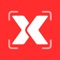# Gauthmath - Talk to tutors now

by GAUTHTECH PTE. LTD.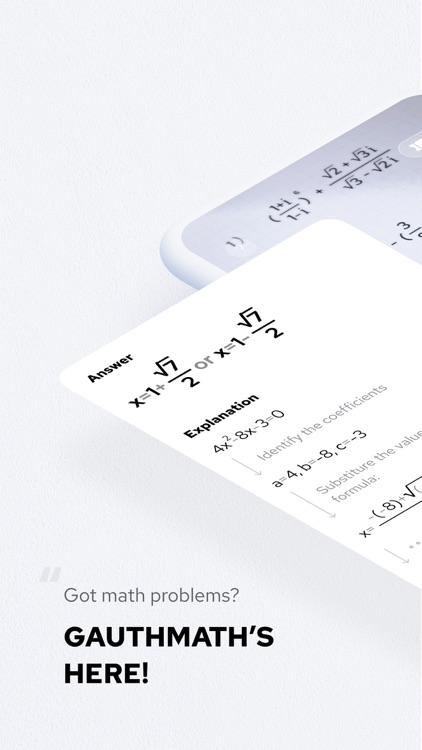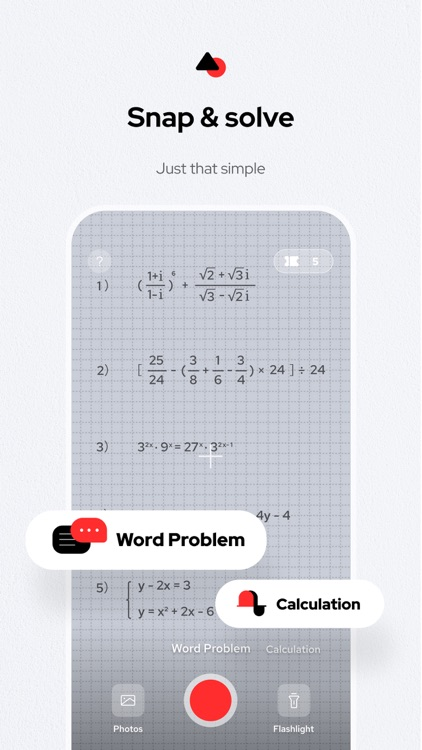Gauthmath is the fastest math problem solver with no cost online real tutors for algebra, graphing, calculus, even word problems, and all other math problems! Simply snap a photo of your question and get step-by-step answers instantly.### App Details

Version
1.5.1
Rating
(1454)
Size
115Mb
Genre
Education Productivity
Last updated
September 13, 2021
Release date
December 12, 2020

### App Screenshots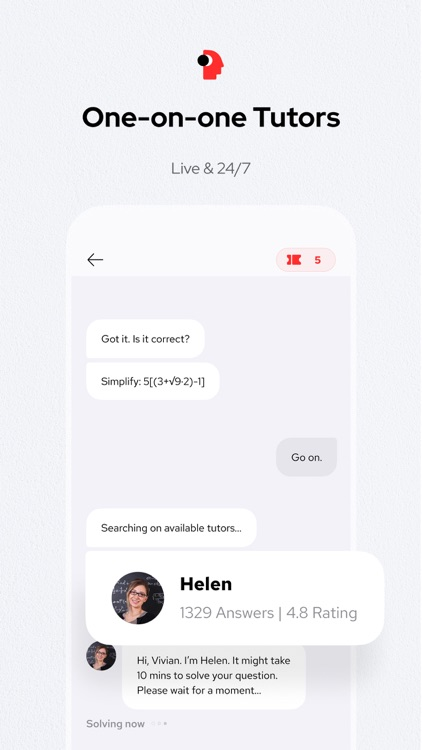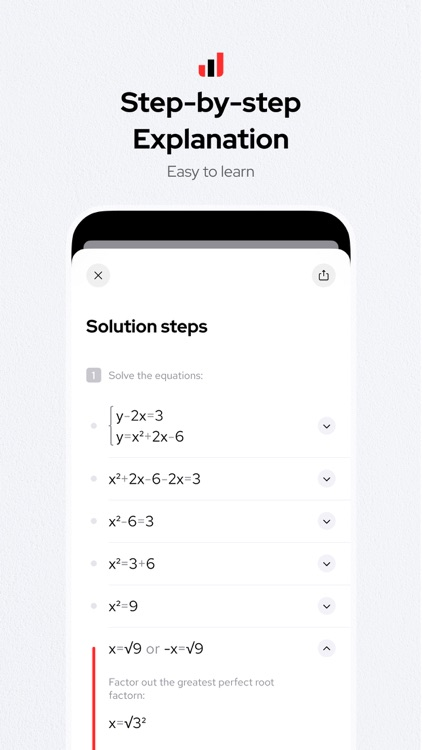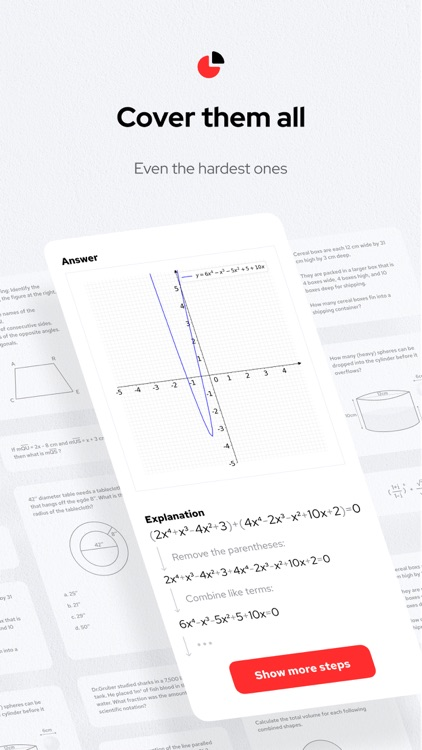### App Store Description

Gauthmath is the fastest math problem solver with no cost online real tutors for algebra, graphing, calculus, even word problems, and all other math problems! Simply snap a photo of your question and get step-by-step answers instantly.

【WORD PROBLEM FULLY COVERED】Gauthmath can solve word problems that others can't. With real tutors online, you can get answers with step-by-step explanations to all your maths questions.

【FREE MATH PROBLEM SOLVER】Gauthmath can help and solve all your math homework, just take a photo or scan your maths homework, Gauthmath will instantly get you the math problem answer!

【MATH ANSWER IN SECONDS】Gauthmath got thousands of helpers and experts, once they got your math problem photo, an answer will be there instantly, and a step-by-step solution will be provided to you if you needed. Just ask them, anywhere, anytime, and even 24/7.

【WORLD'S EASIEST MATH SCANNER】Gauthmath can help you solve all kinds of math problems! Even the hardest types of problems. It covers all kinds of math problems IB/ A Level/ SAT / ACT / AP / GCSE / HSC math and other advanced mathematics.

►MAIN FEATURES
· 100% FREE for all functions!
· Solve math problems instantly with a math expert! Faster and better!
· LIVE Math experts available 24/7, NO LIMIT & FREE!
· Math solutions with step-by-step explanations!
· Gauthmath solves word problems for Math no other tools can!
· Snap a math question, and you will get the problem solved in seconds!

►MATH TOPICS COVERED
·Word problems
·Algebra (Real Number; Arithmetic; Set theory; Expression; Logarithm; Complex Number)
·Function (Linear; Quadratic; Polynomial; Exponential; Rational; Logarithmic; Inverse Function)
·Geometry (Plane & Solid Geometry; Algebra & lines; lines and planes in space; transformation)
·Trigonometry (Trigonometric Ratios; Law of Sines; Law of Cosines; Reciprocal Properties)
·Calculus (Derivatives; Integrals; Limits; the tangent/area problem)
·Statistics and Data Analysis (Probability; data representation; random variables; binomial theorem; Poisson distribution; normal distributions; confidence intervals)
·Matrix (matrix algebra; the system of linear equations and matrices)
·Logic (reasoning and proof; conditional statements; inductive reasoning; deductive reasoning)

►EASIEST WAY OF MATH
You just need to use your phone to type in or take a photo of the question, then you can instantly get answers from our math experts. Totally Free!!! Gauthmath is your free portable tutor, always ready to provide you with homework help and improve your learning efficiency! With Gauthmath，you will find math problems like a piece of cake！

If you have any problems in mathematics, leave it to Gauthmath! Your most reliable math helper will provide the best math answers for your math homework. Our math expert services will prepare high-quality math help for you and make your math homework and exams much easier!

Gauthmath, your fastest and most trustworthy math helper!

【LIKE US and STAY CONNECTED】
►Instagram: https://www.instagram.com/gauthmath.official/